Histogram Method

Measurement Studio 2012 for Visual Studio 2010 Help

Edition Date: August 2012

Part Number: 372636F-01

»View Product InfoDownload Help (Windows Only)

Calculates the histogram of the array. The histogram is the count of the number of elements that fall within a specified set of intervals.

Namespace: NationalInstruments.Analysis.Math

Assembly: NationalInstruments.Analysis.Enterprise (in NationalInstruments.Analysis.Enterprise.dll) Version: 12.0.40.318

Syntax

Visual Basic (Declaration)
<SecurityPermissionAttribute(SecurityAction.Demand, Flags := SecurityPermissionFlag.UnmanagedCode)> _
Public Shared Function Histogram ( _
inputData As Double(), _
histogramBase As Double, _
histogramTop As Double, _
histogramIntervals As Integer, _
<OutAttribute> ByRef centerValues As Double() _
) As Integer()

C#
[SecurityPermissionAttribute(SecurityAction.Demand, Flags = SecurityPermissionFlag.UnmanagedCode)]
public static int[] Histogram(
double[] inputData,
double histogramBase,
double histogramTop,
int histogramIntervals,
out double[] centerValues
)

Parameters

inputData
Type: System.Double []
The input data.
histogramBase
Type: System.Double
The lower bound of the range over which the histogram is computed.
histogramTop
Type: System.Double
The upper bound of the range over which the histogram is computed.
histogramIntervals
Type: System.Int32
The number of elements in the histogram and in the centerValues output array.
centerValues
Type: System.Double [] %
Upon return, contains the mid-point values of the intervals (bins) used to determine the histogram.

Return Value

The discrete histogram of the input array, inputData.

Exceptions

ExceptionCondition
ArgumentException

histogramBase is greater than histogramTop.

-or-

The number of samples in inputData is equal to zero.

-or-

The number of histogramIntervals is less than or equal to zero.

DllNotFoundException The analysis library cannot be found.
EntryPointNotFoundException A required operation in the analysis library cannot be found.

Remarks

The true plot of the histogram of the input array is obtained by plotting histogram versus axis.

The histogram is a frequency count of the number of times that a specified interval, or bin, occurs in the input array. The width of the frequency bin is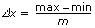where m is the requested number of bins. The centerValues of each bin are set according to the following equation: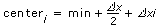Example

If the input array, inputData, is

X = {0, 1, 3, 3, 4, 4, 4, 5, 5, 8}

then the histogram, h(x), of X for eight intervals is

h(X) = {h0, h1, h2, h3, h4, h5, h6, h7} = {1, 1, 0, 2, 3, 2, 0, 1}.

Notice that the histogram of the input array X is a function of X.

This method obtains histogram, h(x), as follows. The method scans the input array X to determine the range of values in it. Then the method establishes the interval width, x, according to the specified number of histogramIntervals,where max represents the maximum value found in the input array X,
min represents the minimum value found in the input array X, and
m represents the specified number of histogramIntervals.

Let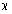represent the output array inputData, because the histogram is a function of X. The method evaluates elements of c using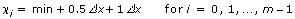The method defines the ith interval to be the range of values from up to but not including,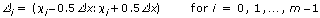and defines the function to be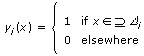The function has unity value if the value of x falls within the specified interval. Otherwise it is zero. Notice that the interval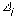is centered about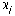, and its width is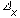.

The last interval,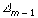, is defined. In other words, if a value is equal to max, it is counted as belonging to the last interval.

Finally, the method evaluates the histogram sequence H using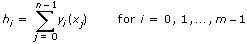where hi represents the elements of the output array histogram, h(x), and
n is the number of elements in the input array X.

Examples

The following example calculates a histogram of the generated noise signal.
VB.NET
Dim inputData(1999) As Double
Dim centerValues(9) As Double
Dim histogramData() As Integer
Dim histogramBase, histogramTop As Double
Dim histogramIntervals As Integer
Dim gaussianNoise As GaussianNoiseSignal = New GaussianNoiseSignal(1.0, 17)

' Generate a Gaussian noise signal that represents inputData
inputData = gaussianNoise.Generate(1000.0, 2000)

histogramIntervals = 10

' Get the minimum and maximum values in the array
histogramBase = ArrayOperation.GetMin(inputData)
histogramTop = ArrayOperation.GetMax(inputData)

' Calculate histogram
histogramData = Statistics.Histogram(inputData, histogramBase, histogramTop, histogramIntervals, centerValues)
C#
double[] inputData = new double;
double[] centerValues = new double;
int[] histogramData;
double histogramBase, histogramTop;
int histogramIntervals;
GaussianNoiseSignal gaussianNoise = new GaussianNoiseSignal(1.0, 17);

// Generate a Gaussian noise signal that represents inputData
inputData = gaussianNoise.Generate(1000.0, 2000);

histogramIntervals = 10;

// Get the minimum and maximum values in the array
histogramBase = ArrayOperation.GetMin(inputData);
histogramTop = ArrayOperation.GetMax(inputData);

// Calculate histogram
histogramData = Statistics.Histogram(inputData, histogramBase, histogramTop, histogramIntervals, out centerValues);